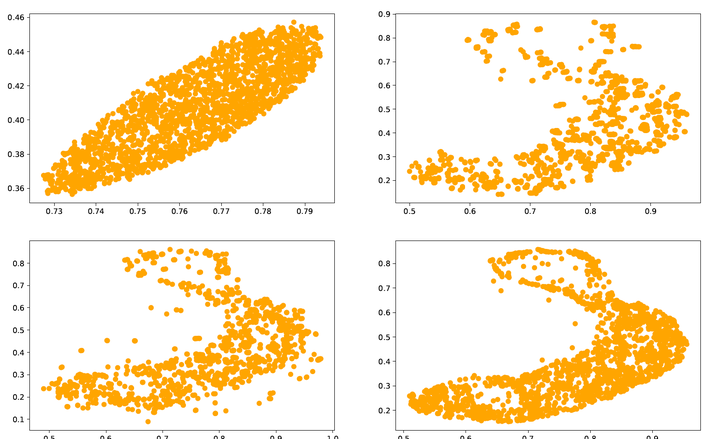# Stability of Semi-Dual Unbalanced Optimal Transport: fast statistical rates and convergent algorithm### Abstract

In this paper, we derive stability results for the semi-dual formulation of unbalanced optimal transport. From a statistical point of view, the gain of stability with respect to the balanced case allows to employ localization arguments while only assuming strong convexity of potentials and recover superparametric rates. Then we derive a provably convergent theoretical algorithm to minimize the semi-dual: if the potentials are constrained to be strongly convex, both the values and minimizers converge at a $1/k$ rate. Under an additional smoothness assumption, the convergence is exponential in the balanced case. Finally we instantiate a tractable version of our theoretical algorithm in the case of strongly convex, possibly smooth potentials. We benchmark the method in the balanced case on a 2D experiment and in the unbalanced case on a medium dimension synthetic experiment.

Type
Publication
ICML 2023